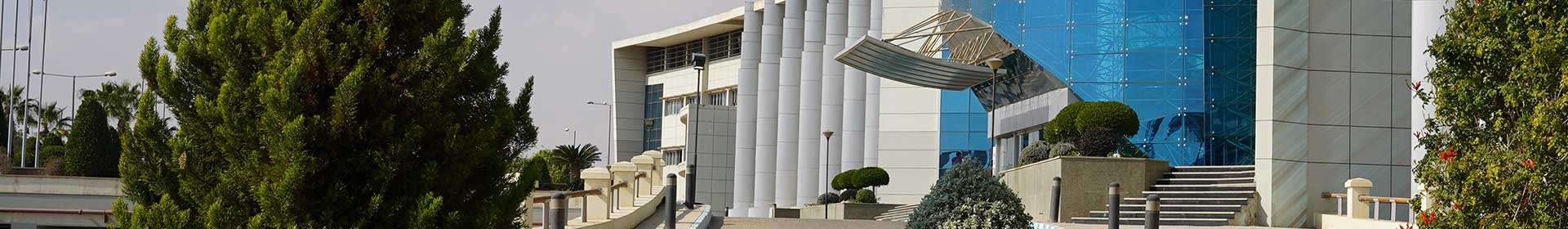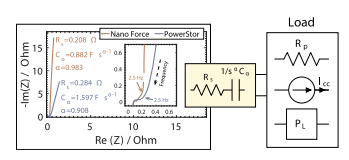Journal

# Supercapacitor discharge under constant resistance, constant current and constant power loads

By
Fouda M.E.
Allagui A.
Elwakil A.S.
Eltawil A.
Kurdahi F.

Supercapacitors, which are now widely used as power sources in various applications, are discharged with one of the following three basic discharge modes: a constant current load, a constant resistance load or a constant power load. A constant current load is one which varies its internal resistance to achieve a constant current regardless of the applied voltage. For the constant resistance case, it results in a change of power as the voltage level changes. And for a constant power load, the load varies its impedance as the input voltage changes in order to keep the power constant. However, explicit solutions to the electrical behavior of supercapacitors under these loads have not been reported yet, particularly by taking into account their fractional-order dynamics. In this work, we derive the voltage, current, power and energy expressions when a supercapacitor is discharging through these three types of loads. A model consisting of a resistance in series with a fractional-order capacitor, also known as constant phase element (CPE), is used. We verify the validity of our expressions via experimental results on commercial devices and provide a critical comparison with the integer-order Rs -C model. © 2019 Elsevier B.V.